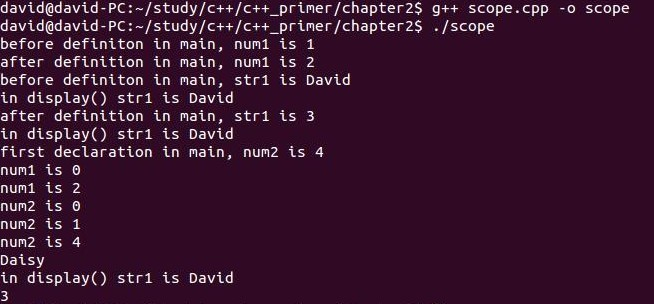# C++ 变量作用域小探索

``` #include ```

``` int num1 = 1; std::string str1 = "David"; //display the value of str1 void display(); ```

```int main() { std::cout << "before definiton in main, num1 is " << num1 << std::endl; int num1 = 2; std::cout << "after definition in main, num1 is " << num1 << std::endl; std::cout << "before definiton in main, str1 is " << str1 << std::endl; display(); int str1 = 3; std::cout << "after definition in main, str1 is " << str1 << std::endl; display(); int num2 = 4; std::cout << "first declaration in main, num2 is " << num2 << std::endl; //std::string num2 = "Daisy"; //compile error:conflicting declaration //int num2 = 5; //compile error:redeclaration //statement scope for(int num1 = 0, sum = 0; num1 < 1; num1++) { sum += num1; std::cout << "num1 is " << num1 << std::endl; } std::cout << "num1 is " << num1 << std::endl; //statement scope for(int num2 = 0, sum = 0; num2 < 2; num2++) { sum += num2; std::cout << "num2 is " << num2 << std::endl; } std::cout << "num2 is " << num2 << std::endl; //block scope { std::string str1 = "Daisy"; std::cout << str1 << std::endl; display(); } std::cout << str1 << std::endl; } void display() { std::cout << "in display() str1 is " << str1 << std::endl; } ```1.首先对于num1这个变量，已经在第三行定义成了全局变量并初始化为1，然后在main函数里第11行，打印输出很显然就是

“before definiton in main, num1 is 1”

"after definition in main, num1 is 2"

2.对于在第四行第一次定义的string变量 str1，赋值为“David”。在main函数里第15行打印一下，很显然结果是

"before definiton in main, str1 is David"， 就如同结果图片里面的第三行输出。

"in display() str1 is David"

“after definition in main, str1 is 3”，然后我又调用了一下displays()函数，想看看是不是全局变量的也改变了，打印结果是

"in display() str1 is David"，说明全局变量并没有被改变！

3.在main函数里第21行，定义了一个int变量num2，赋值为4，然后打印一下。结果很显然是

"first declaration in main, num2 is 4"

4.首先在一个循环里面，重新定义一下num1，我想，既然可以从“外”往“里”屏蔽，那么从“里”往“里的里”也应该能屏蔽，果然，在for里面，num1的值是在语句作用域里的值，跳出循环以后，再打印就是函数作用域里的num1值了（不是全局的值，它已经被main里的屏蔽，这点从打印的是2 而不是 1就看得出来）

This site uses Akismet to reduce spam. Learn how your comment data is processed.